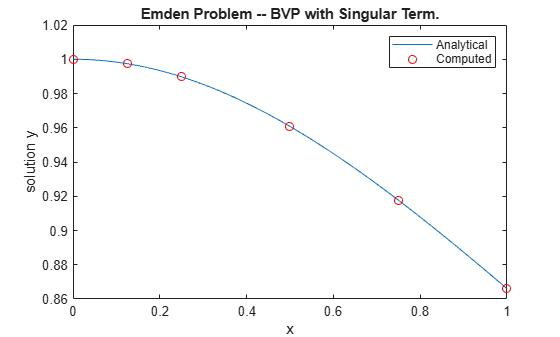# Solve BVP with Singular Term

This example shows how to solve Emden's equation, which is a boundary value problem with a singular term that arises in modeling a spherical body of gas.

After reducing the PDE of the model using symmetry, the equation becomes a second-order ODE defined on the interval $\left[0,1\right]$,

${y}^{\prime \prime }+\frac{2}{x}{y}^{\prime }+{y}^{5}=0$.

At $\mathit{x}=0$, the $\left(2/\mathit{x}\right)$ term is singular, but symmetry implies the boundary condition ${\mathit{y}}^{\prime }\left(0\right)=0$. With this boundary condition, the term $\left(2/\mathit{x}\right){\mathit{y}}^{\prime }$ is well defined as $\mathit{x}\to 0$. For the boundary condition $\mathit{y}\left(1\right)=\sqrt{3}/2$, the BVP has an analytical solution that you can compare to a numeric solution calculated in MATLAB®,

$y\left(x\right)={\left[\sqrt{1+\frac{{x}^{2}}{3}}\right]}^{-1}$.

The BVP solver `bvp4c` can solve singular BVPs that have the form

${y}^{\prime }=S\frac{y}{x}+f\left(x,y\right)$.

The matrix $\mathit{S}$ must be constant and the boundary conditions at $\mathit{x}=0$ must be consistent with the necessary condition $\mathit{S}\cdot \mathit{y}\left(0\right)=0$. Use the `'SingularTerm'` option of `bvpset` to pass the `S` matrix to the solver.

You can rewrite Emden's equation as a system of first-order equations using ${\mathit{y}}_{1}=\mathit{y}$ and ${\mathit{y}}_{2}={\mathit{y}}^{\prime }$ as

${{\mathit{y}}_{1}}^{\prime }={\mathit{y}}_{2}$,

${{\mathit{y}}_{2}}^{\prime }=-\frac{2}{\mathit{x}}{\mathit{y}}_{2}-{\mathit{y}}_{1}^{5}$.

The boundary conditions become

${\mathit{y}}_{2}\left(0\right)=0$,

${\mathit{y}}_{1}\left(1\right)=\sqrt{3}/2$.

In the required matrix form, the system is

$\left[\begin{array}{c}{{\mathit{y}}_{1}}^{\prime }\text{\hspace{0.17em}}\\ {{\mathit{y}}_{2}}^{\prime }\end{array}\right]=\frac{1}{\mathit{x}}\left[\begin{array}{cc}0& 0\\ 0& -2\end{array}\right]\left[\begin{array}{c}{\mathit{y}}_{1}\\ {\mathit{y}}_{2}\end{array}\right]+\left[\begin{array}{c}{\mathit{y}}_{2}\\ -{\mathit{y}}_{1}^{5}\end{array}\right]$.

In matrix form it is clear that $\mathit{S}=\left[\begin{array}{cc}0& 0\\ 0& -2\end{array}\right]$ and $\mathit{f}\left(\mathit{x},\mathit{y}\right)=\left[\begin{array}{c}{\mathit{y}}_{2}\\ -{\mathit{y}}_{1}^{5}\end{array}\right]$.

To solve this system of equations in MATLAB, you need to code the equations, boundary conditions, and options before calling the boundary value problem solver `bvp4c`. You either can include the required functions as local functions at the end of a file (as done here), or save them as separate, named files in a directory on the MATLAB path.

### Code Equation

Create a function to code the equations for $\mathit{f}\left(\mathit{x},\mathit{y}\right)$. This function should have the signature `dydx = emdenode(x,y)`, where:

• `x` is the independent variable.

• `y` is the dependent variable.

• `dydx(1)` gives the equation for ${{\mathit{y}}_{1}}^{\prime }$, and `dydx(2)` the equation for ${{\mathit{y}}_{2}}^{\prime }$.

These inputs are automatically passed to the function by the solver, but the variable names determine how you code the equations. In this case:

```function dydx = emdenode(x,y) dydx = [y(2) -y(1)^5]; end ```

The term that contains `S` is passed to the solver using options, so that term is not included in the function.

### Code Boundary Conditions

Now, write a function that returns the residual value of the boundary conditions at the boundary points. This function should have the signature `res = emdenbc(ya,yb)`, where:

• `ya` is the value of the boundary condition at the beginning of the interval.

• `yb` is the value of the boundary condition at the end of the interval.

For this problem, one of the boundary conditions is for ${\mathit{y}}_{1}$, and the other is for ${\mathit{y}}_{2}$. To calculate the residual values, you need to put the boundary conditions into the form $\mathit{g}\left(\mathit{x},\mathit{y}\right)=0$.

In this form the boundary conditions are

${\mathit{y}}_{2}\left(0\right)=0$,

${\mathit{y}}_{1}\left(1\right)-\sqrt{3}/2=0$.

The corresponding function is then

```function res = emdenbc(ya,yb) res = [ya(2) yb(1) - sqrt(3)/2]; end ```

### Create Initial Guess

Lastly, create an initial guess of the solution. For this problem, use a constant initial guess that satisfies the boundary conditions, and a simple mesh of five points between 0 and 1. Using many mesh points is unnecessary since the BVP solver adapts these points during the solution process.

${\mathit{y}}_{1}=\sqrt{3}/2$,

${\mathit{y}}_{2}=0$.

```guess = [sqrt(3)/2; 0]; xmesh = linspace(0,1,5); solinit = bvpinit(xmesh, guess);```

### Solve Equation

Create a matrix for `S` and pass it to `bvpset` as the value of the `'SingularTerm'` option. Finally, call `bvp4c` with the ODE function, boundary condition function, initial guess, and option structure.

```S = [0 0; 0 -2]; options = bvpset('SingularTerm',S); sol = bvp4c(@emdenode, @emdenbc, solinit, options);```

### Plot Solution

Calculate the value of the analytical solution in $\left[0,1\right]$.

```x = linspace(0,1); truy = 1 ./ sqrt(1 + (x.^2)/3);```

Plot the analytical solution and the solution calculated by `bvp4c` for comparison.

```plot(x,truy,sol.x,sol.y(1,:),'ro'); title('Emden Problem -- BVP with Singular Term.') legend('Analytical','Computed'); xlabel('x'); ylabel('solution y');```### Local Functions

Listed here are the local helper functions that the BVP solver `bvp4c` calls to calculate the solution. Alternatively, you can save these functions as their own files in a directory on the MATLAB path.

```function dydx = emdenode(x,y) % equation being solved dydx = [y(2) -y(1)^5]; end %------------------------------------------- function res = emdenbc(ya,yb) % boundary conditions res = [ya(2) yb(1) - sqrt(3)/2]; end %-------------------------------------------```